Next: Variance of the estimator Up: Deriving the Optimal Scoring Previous: Deriving the Optimal Scoring

## Expected value of the estimator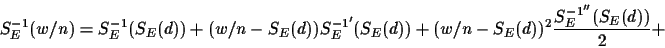O( (w/n - SE(d))3)

Simplifying SE-1 and its derivatives we can rewrite the above equation as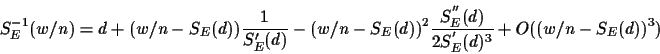Taking expected values we find that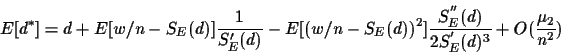since E[w/n - SE(d)] = E[w/n] - SE(d) = 0. When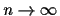then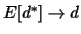, and hence E[d*] is a consistent estimator (we could correct it to any order of n if desired).

Chantal Korostensky
1999-07-14